# The aftermath of ORF523 and the final

It has been two weeks since my last post on the blog, and I have to admit that it felt really good to take a break from my 2-posts-per-week regime of the previous months. Now that ORF523 is over the blog will probably be much more quiet in the near future, though I have a few cool topics that I want to discuss in the coming weeks and I’m also hoping to get interesting guest posts. In any case the next period of intense activity will probably be next Fall, as I will be visiting the newly created Simons Institute for the Theory of Computing at UC Berkeley and I plan to blog about the new tricks that I will learn from the program on the Theoretical Foundations of Big Data Analysis and the one on Real Analysis in Computer Science. More details on this to come soon!

For those of you who followed assiduously my lectures I thought that you might want to take a look the final that I gave to my students this morning. The first part is based on this paper by Alon and Naor, and the second part is based on this paper by Nesterov (how could I not finish with a result from Nesterov??). Enjoy!

An SDP relaxation based on Grothendieck’s inequality

In this problem we consider the space of rectangular matricesfor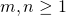. We denote by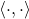the Frobenius inner product on, that is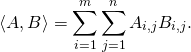Let. We consider the following norm on: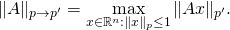In other words this is the operator norm ofwhen we view it as a mapping fromto. In this problem we are interested in computing this operator norm for a given matrix.

1. Is the problem of computing the operator norm ofa convex problem?

2. For what values of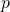and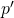can you easily propose a polynomial-time algorithm?

3. Let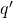be such that. Recall why one hasWe will now focus on the caseand, that isWe also consider the following quantity: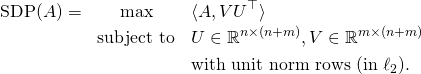4. Show that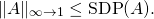5. Show that the problem of computing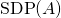for a given matrixis an SDP.

Grothendieck’s inequality says that there exists a constantsuch that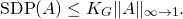6. What is the implication of Grothendieck’s inequality for the problem of computing the norm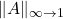?

Coordinate Descent

Letbe a differentiable convex function on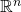that admits a minimizer. We denote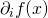for the partial derivative in directionofat(it is the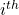coordinate of). In this problem we consider the Coordinate Descent algorithm to solve the problem: Let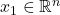and for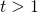letWe say thatis coordinate-wise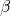-smooth if for any,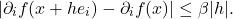1. Is coordinate-wise smoothness a stronger or weaker assumption than standard smoothness?

2. Assume thatis coordinate-wise-smooth and let. Show first that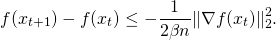Let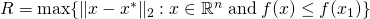, show next that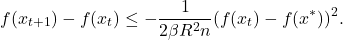Conclude by proving that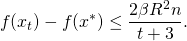Hint: to deal with the term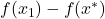you can first show thathas to be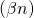-smooth.

3. Can you use this algorithm and the convergence result described above to show existence of sparse minimizers in the spirit of what we did with the Conditional Gradient Descent algorithm?

4. In general how does the computational complexity of Coordinate Descent compares to the computational complexity of Gradient Descent to attain an-optimal point for a-smooth function? What happens if you assume access to an oracle to compute?

5. Same question but in the special case wherewithand.

6. Can you propose a variant of Coordinate Descent where the computation ofwould be much faster and yet this newwould still allow us to prove the rate of convergence of question 2.? Hint: use randomization!

1. 67, 1.79
This entry was posted in Optimization. Bookmark the permalink.